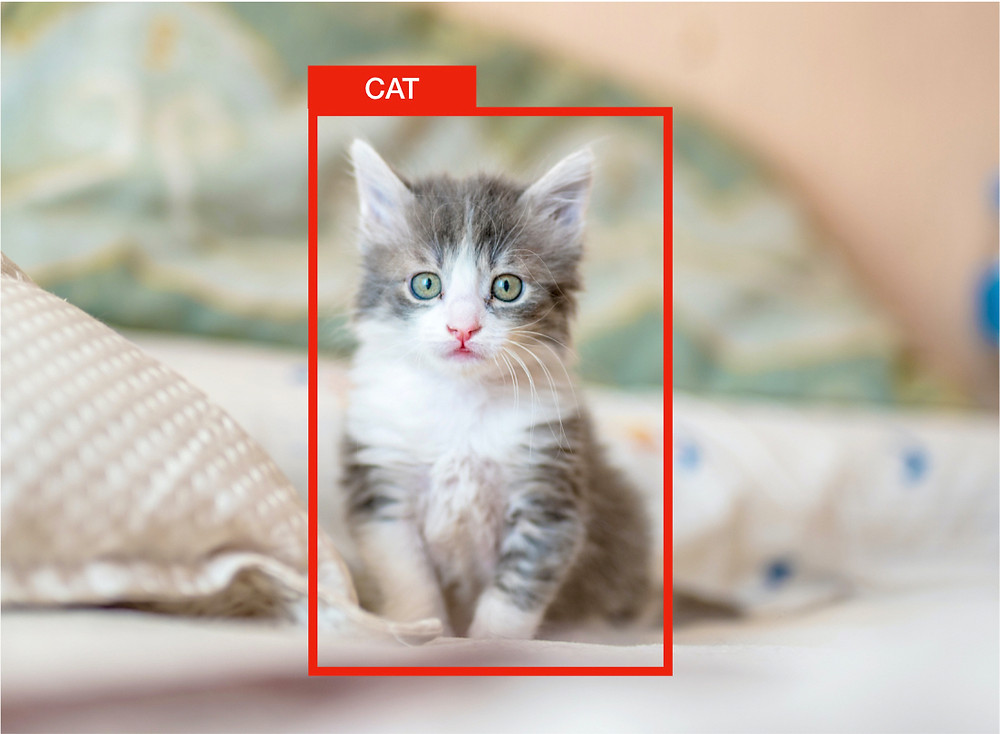Search
• Shivy Yohanandan

# mAP (mean Average Precision) might confuse you!One can be forgiven for taking mAP (mean average precision) to literally mean the average of precisions. Nevertheless, you couldn’t be further from the truth!

Let me explain.

In computer vision, mAP is a popular evaluation metric used for object detection (i.e. localisation and classification). Localization determines the location of an instance (e.g. bounding box coordinates) and classification tells you what it is (e.g. a dog or cat).Image classification and localization

Many object detection algorithms, such as Faster R-CNN, MobileNet SSD, and YOLO, use mAP to evaluate their models for publishing their research.

You might ask, if it’s such a popular metric, why is it still confusing?

Fair enough!

mAP stands for Mean Average Precision (as you might already have guessed looking at the title).

You might think it is the average of the Precision.

If you do not know already:

Precision measures how accurate your predictions are. i.e. the percentage of your predictions are correct.

It measures how many of the predictions that your model made were actually correct.TP = True Positives (Predicted as positive as was correct) FP = False Positives (Predicted as positive but was incorrect)

If that was the case, let’s calculate mAP for the following image:

Object detection example for Advanced driver-assistance systems (ADAS)From the image, we get:Image classification and localization

True Positives (TP) = 1 Fasle Positives (FP) = 0Because we only have one value the average of precision will be 1.

Looking at the mAP score, you might end-up using this model in your application. That would be a disaster.

AND THAT’S THE CATCH! DON’T LET THE TERM MISLEAD YOU.

mAP is not calculated by taking the average of precision values.

Object detection systems make predictions in terms of a bounding box and a class label.Object detection example detecting a cat (Original cat Photo by Kote Puerto on Unsplash)

For each bounding box, we measure overlap between the predicted bounding box and the ground truth bounding box. This is measured by IoU (intersection over union).For object detection tasks, we calculate Precision and Recall using IoU value for a given IoU threshold.

For example, if IoU threshold is 0.5, and the IoU value for a prediction is 0.7, then we classify the prediction as True Positive (TF). On the other hand, if IoU is 0.3, we classify it as False Positive (FP).That also means that for a prediction, we may get different binary TRUE or FALSE positives, by changing the IoU threshold.Another important term to understand is Recall.

Recall measures how well you find all the positives. For example, we can find 80% of the possible positive cases in our top K predictions.TP = True Positives (Predicted as positive as was correct) FN = False Negatives (Failed to predict an object that was there)

The general definition for the Average Precision (AP) is finding the area under the precision-recall curve above.
mAP (mean average precision) is the average of AP.

In some contexts, AP is calculated for each class and averaged to get the mAP. But in others, they mean the same thing. For example, for COCO challenge evaluation, there is no difference between AP and mAP.

AP is averaged over all categories. Traditionally, this is called “mean average precision” (mAP). We make no distinction between AP and mAP (and likewise AR and mAR) and assume the difference is clear from context. COCO Evaluation

The mean Average Precision or mAP score is calculated by taking the mean AP over all classes and/or overall IoU thresholds, depending on different detection challenges that exist.

For example:

In PASCAL VOC2007 challenge, AP for one object class is calculated for an IoU threshold of 0.5. So the mAP is averaged over all object classes.

For the COCO 2017 challenge, the mAP is averaged over all object categories and 10 IoU thresholds.

So for the above ADAS image, let’s calculate the mAP using the actual formula: Here we assume that the confidence score threshold is 0.5 and the IoU threshold is also 0.5.

So we calculate the AP at IoU threshold o.5.

For simplicity, we will calculate an average for the 11-point interpolated AP. In the latest research, more advanced techniques have been introduced to calculate the AP.

True Positives (TP) = 1 Fasle Positives (FP) = 0 False Negatives (FN) = 1We plot the 11 points interpolated Precision-Recall curve.Precision-Recall Curve

We now calculate AP by taking the area under the PR curve. This is done by segmenting the recalls evenly to 11 parts: {0,0.1,0.2,…,0.9,1}.So mAP@0.5 for the image is 0.545, not 1.

Hope this clarifies your misunderstanding on mAP.

Check-out this post for more details on creating a robust object detection model.

References:

Van Etten, A. (2019, January). Satellite imagery multiscale rapid detection with windowed networks. In 2019 IEEE Winter Conference on Applications of Computer Vision (WACV) (pp. 735–743). IEEE.

Authors

Shivy Yohanandan Sabina Pokhrel